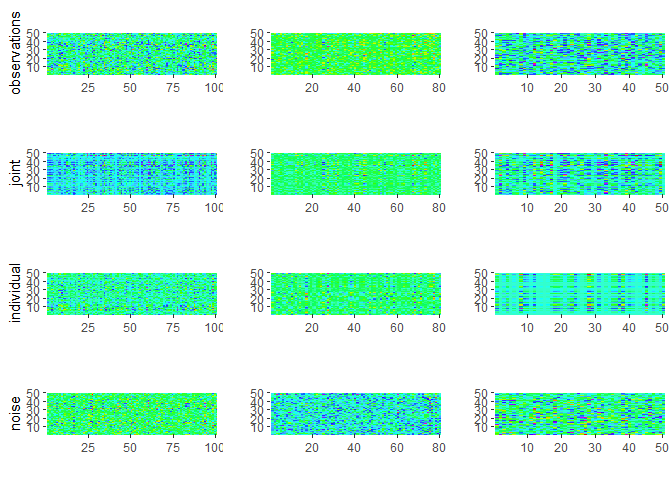# RaJIVE

RaJIVE (Robust Angle based Joint and Individual Variation Explained) is a robust alternative to the aJIVE method for the estimation of joint and individual components in the presence of outliers in multi-source data. It decomposes the multi-source data into joint, individual and residual (noise) contributions. The decomposition is robust with respect to outliers and other types of noises present in the data.

## Installation

You can install the released version of RaJIVE from CRAN with:

``install.packages("RaJIVE")``

And the development version from GitHub with:

``````# install.packages("devtools")
devtools::install_github("ericaponzi/RaJIVE")``````

## Example

This is a basic example which shows how to use RaJIVE on simple simulated data:

### Running robust aJIVE

``````library(RaJIVE)
## basic example code
n <- 50
pks <- c(100, 80, 50)
Y <- ajive.data.sim(K =3, rankJ = 3, rankA = c(7, 6, 4), n = n,
pks = pks, dist.type = 1)

initial_signal_ranks <-  c(7, 6, 4)
data.ajive <- list((Y\$sim_data[]), (Y\$sim_data[]), (Y\$sim_data[]))
ajive.results.robust <- Rajive(data.ajive, initial_signal_ranks)``````

The function returns a list containing the aJIVE decomposition, with the joint component (shared across data sources), individual component (data source specific) and residual component for each data source.

### Visualizing results:

• Joint rank:
``````
get_joint_rank(ajive.results.robust)
#>  3``````
• Individual ranks:
``````get_individual_rank(ajive.results.robust, 1)
#>  5
get_individual_rank(ajive.results.robust, 2)
#>  3
get_individual_rank(ajive.results.robust, 3)
#>  1``````
• Heatmap decomposition:
``decomposition_heatmaps_robustH(data.ajive, ajive.results.robust)``• Proportion of variance explained:
``````showVarExplained_robust(ajive.results.robust, data.ajive)
#> \$Joint
#>  0.3148569 0.3349692 0.4197429
#>
#> \$Indiv
#>  0.5499653 0.4156423 0.1522468
#>
#> \$Resid
#>  0.1351778 0.2493886 0.4280103``````